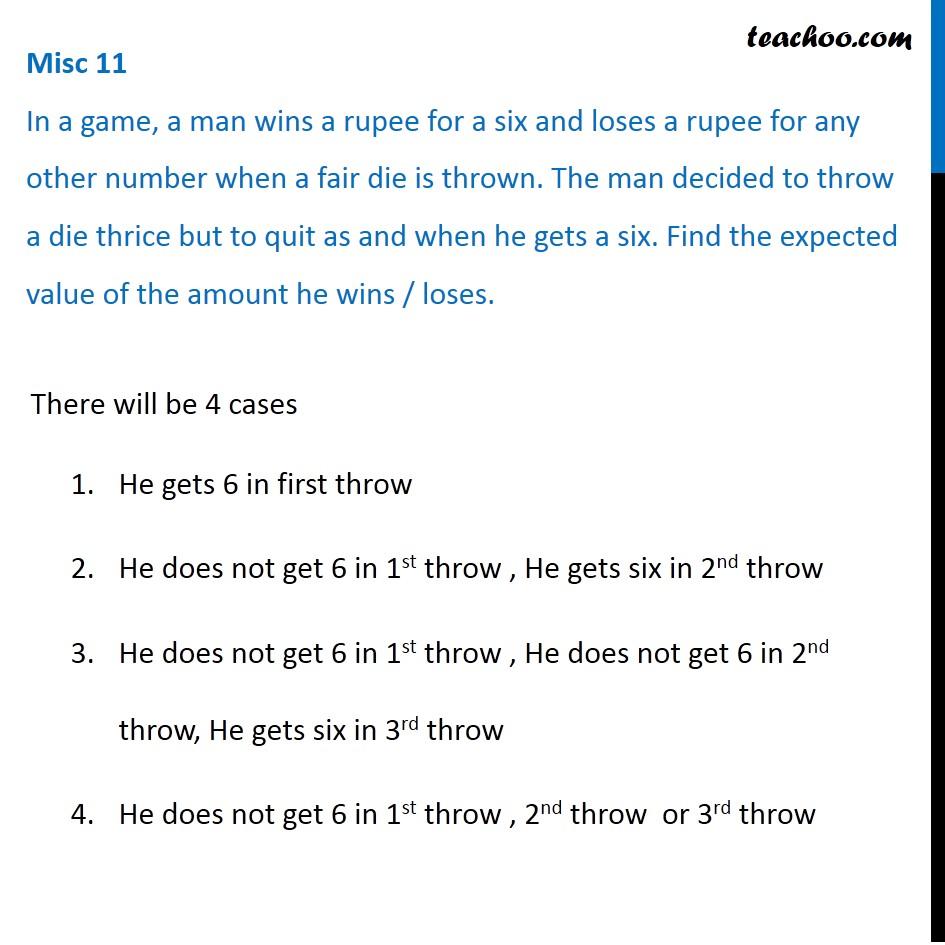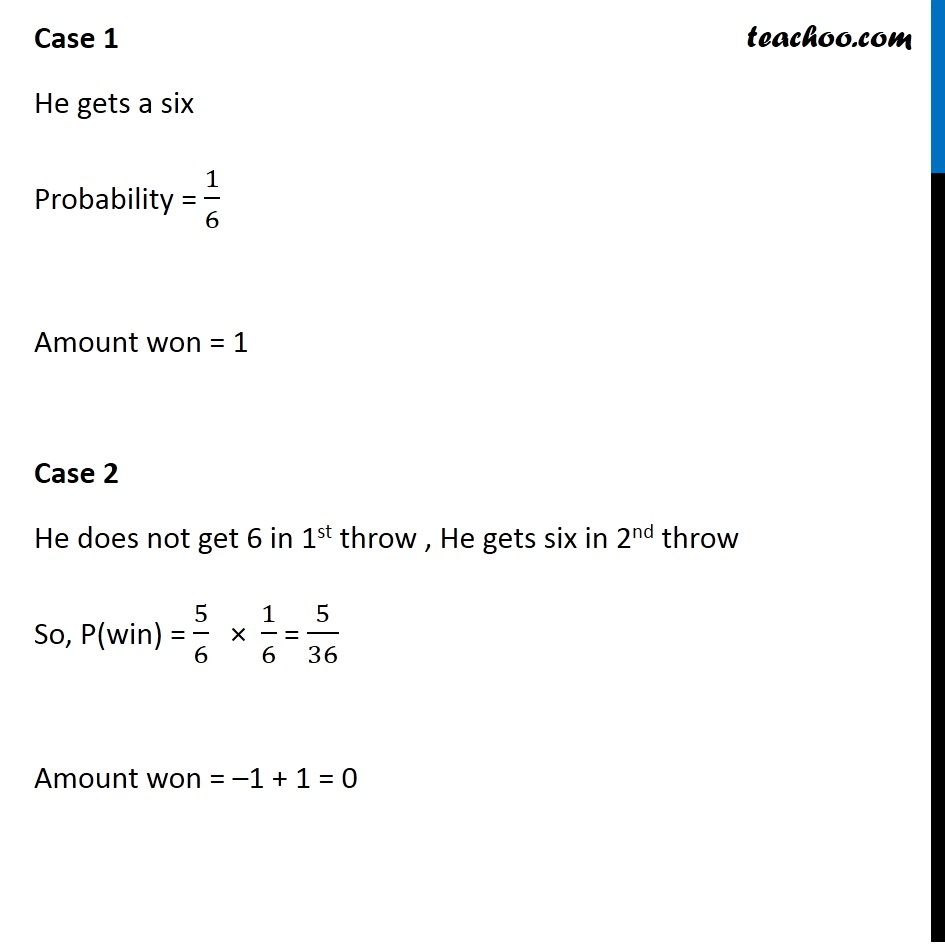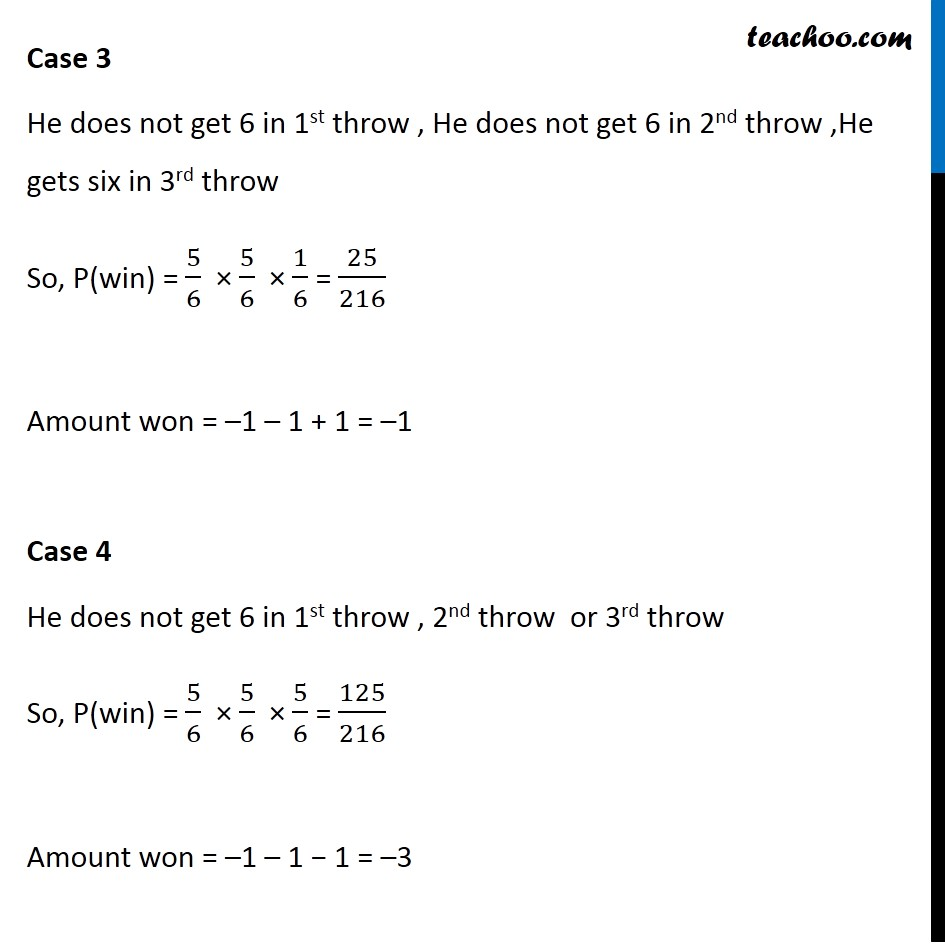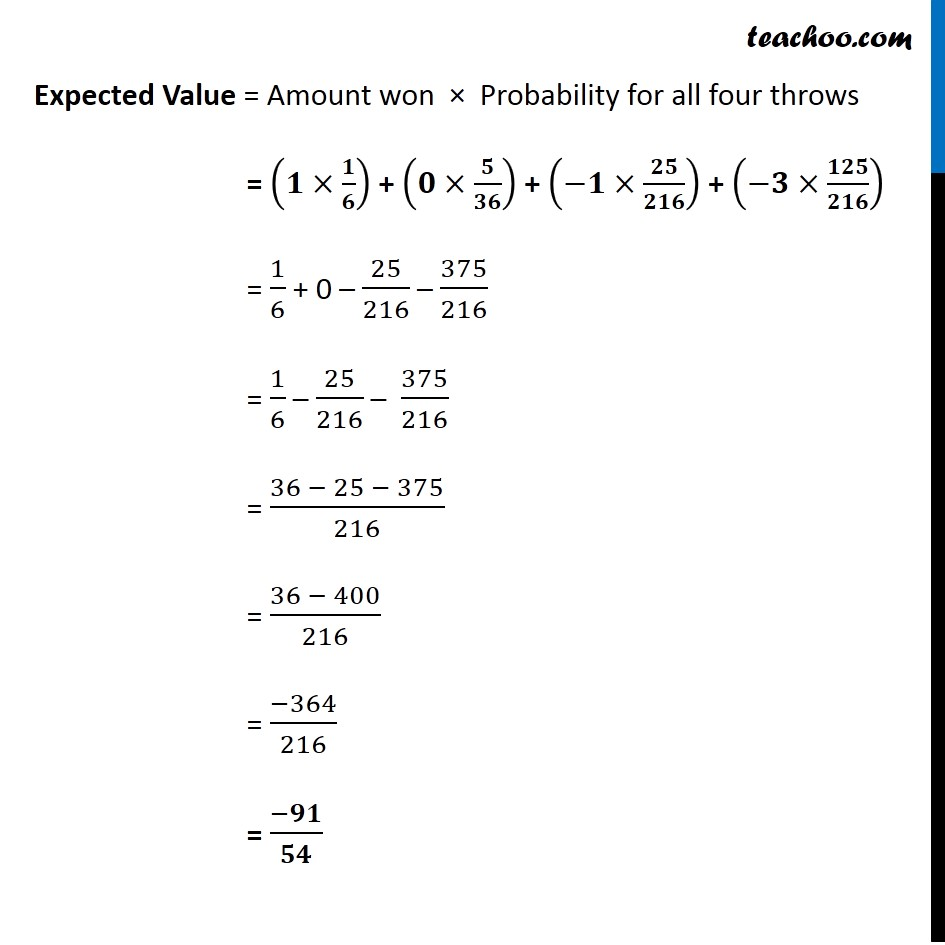Miscellaneous

Chapter 13 Class 12 Probability
Serial order wiseGet live Maths 1-on-1 Classs - Class 6 to 12

### Transcript

Misc 11 In a game, a man wins a rupee for a six and loses a rupee for any other number when a fair die is thrown. The man decided to throw a die thrice but to quit as and when he gets a six. Find the expected value of the amount he wins / loses.There will be 4 cases He gets 6 in first throw He does not get 6 in 1st throw , He gets six in 2nd throw He does not get 6 in 1st throw , He does not get 6 in 2nd throw, He gets six in 3rd throw He does not get 6 in 1st throw , 2nd throw or 3rd throw Case 1 He gets a six Probability = 1/6 Amount won = 1 Case 2 He does not get 6 in 1st throw , He gets six in 2nd throw So, P(win) = 5/6 × 1/6 = 5/36 Amount won = –1 + 1 = 0 Case 3 He does not get 6 in 1st throw , He does not get 6 in 2nd throw ,He gets six in 3rd throw So, P(win) = 5/6 × 5/6 × 1/6 = 25/216 Amount won = –1 – 1 + 1 = –1 Case 4 He does not get 6 in 1st throw , 2nd throw or 3rd throw So, P(win) = 5/6 × 5/6 × 5/6 = 125/216 Amount won = –1 – 1 − 1 = –3 Expected Value = Amount won × Probability for all four throws = (𝟏×𝟏/𝟔) + (𝟎×𝟓/𝟑𝟔) + (−𝟏×𝟐𝟓/𝟐𝟏𝟔) + (−𝟑×𝟏𝟐𝟓/𝟐𝟏𝟔) = 1/6 + 0 – 25/216 – 375/216 = 1/6 – 25/216 – 375/216 = (36 − 25 − 375)/216 = (36 − 400)/216 = (−364)/216 = (−𝟗𝟏)/𝟓𝟒